Learn to Code via Tutorials on Repl.it!

← Back to all posts

# Introduction

As much as I like to wake up each morning and think that computers work through the means of magic or some seagulls with keyboards, the harsh reality is that they don't. My aim throughout this tutorial will be to give you as much insight as I possibly can about the inner workings of a computer while still making it simple to understand for a normal person. Here is what you can expect from this tutorial:

• Circuits
• Binary
• Logic Gates

As for later in the tutorial where I'll be explaining logic gates, I recommend you follow along with my examples through the use of a simulator like CircuitVerse or my own which lacks a user interface but still works for prototyping small circuits. In all honesty, my logic simulator is probably a lot worse and was just something that I made in 5 minutes as a supplement to this tutorial.

Disclaimer: I would like to note that this tutorial won't be teaching any specific code or anything like that but will rather be giving a general overview of some concepts as well as a few diagrams.

# Circuits

### Basic Circuits

On the lowest of levels, computers are essentially just a smart configuration of some wires which are able to handle and interpret data. When these wires are combined together with a power source and an output they create what is known as an electrical circuit. If you recall from science class, circuits often need switches as inputs that can control the output of a circuit something like this: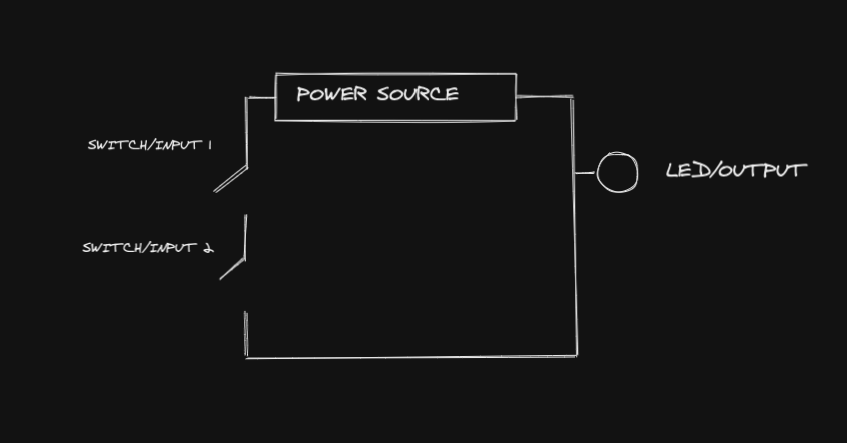Note: I chose to leave out components such as resistors for simplicity

In the image above, you can see that the LED (output) is off because the current can not flow from the power source due to the open switches. Based on this diagram, if you were to close either one of the switches, the LED would still remain off as the current would be cut by the other switch. So the only scenario in which the LED would be powered is when both switches are closed, which is called an and gate (I will get into this later on).

### Transistors

Switches come with one major issue though, they are slow. Back in the early days of computing, computers used to use switches controlled by electromagnets which tended to be slow and had a high chance of getting jammed. Then came fancier new things such as vacuum tubes, but they were still incredibly slow considering the speed of computers today. This speed issue was finally solved by a component known as a transistor which functioned like a switch except it was opened and closed by an electrical component. Here is what a transistor looks like: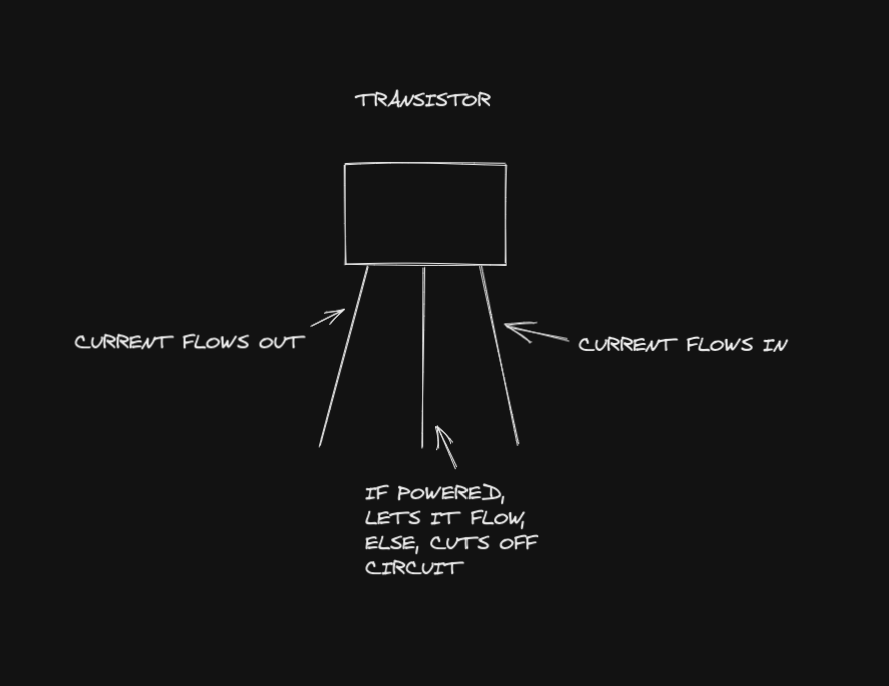Here you can see how the transistor has three separate wires with the middle one controlling the state of the transistor. Just to give you an idea of how important transistors are in modern-day CPUs, the Intel i7 is estimated to have around 4 billion transistors. Transistors such as these can be used to construct logic gates on breadboards or be soldered into a circuit board as a switch. In the context of this tutorial, transistors won't be too important but I thought it would be appropriate to at least have a small section covering them.

Now that basic circuits are covered, let's move on to something a bit more interesting like a binary (No pun intended, but get in? Bit and binary).

# Binary

### Binary's Meaning

I can assume that most of you have heard of binary and know that it consists of 1's and 0's but in this section, I will be going slightly more in-depth into what it actually means. Believe it or not, binary is an abstraction of voltages which means that 1's and 0's are actually meaningless to a computer. We as humans use 1's to represent a high voltage (flowing current) and 0's to represent the opposite, a low voltage (no current). So when someone says input is 1, they actually mean that the input has power and is on.

### Base 2 Number System

Binary runs on what is known as the base 2 number system. To give some context, the number system that is mainly used is called the base 10 number system meaning that there are 10 digits. For example, you would count, zero, one, two, three, four, five, six, seven, eight, nine, but then would run out of digits and increment the next place value to a 1 and reset the current one. This number now is represented as one-zero, or ten as we call it.

The base 2 number system follows this exact concept but with only 2 digits as opposed to 10. An example of counting to 10 in base 2 would look like this:

``````zero(0)
one(1)
one-zero(2)
one-one(3)
one-zero-zero(4)
one-zero-one(5)
one-one-zero(6)
one-one-one(7)
one-zero-zero-zero(8)
one-zero-zero-one(9)
one-zero-one-zero(10)``````

Note: This is a general computer tutorial and not a binary one so I will only be going into addition as opposed to other arithmetic operations

Based on what we learned in the last section, we can apply that knowledge to add two numbers together in binary. Here is a small example of adding the numbers, 7 and 4: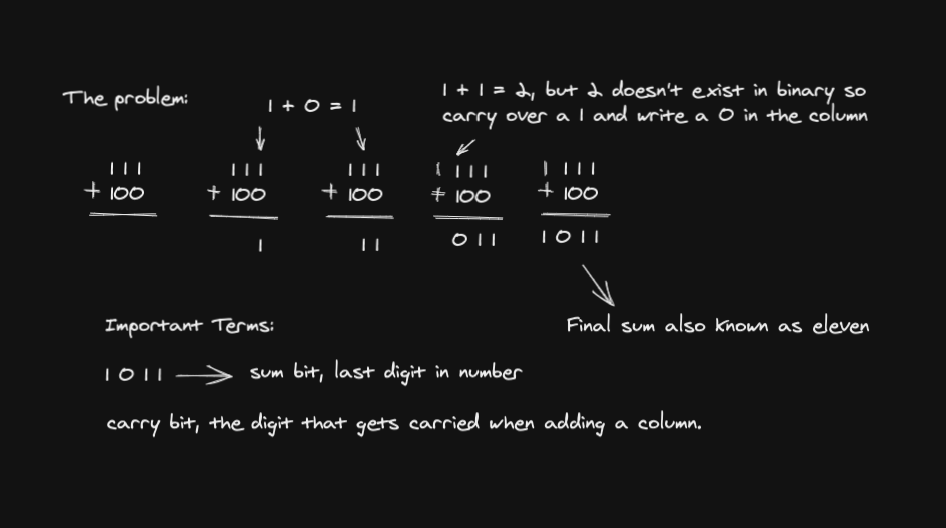In the example above, you can see that 7 and 4 are added just how you would in the base 10 number system and that you carry over a digit or bit whenever the place value needs to be incremented. There are also some important terms to know, such as sum bit which is the bit with the least place value or the rightmost one. Another term is the carry bit which as the name states is the bit that gets carried over when adding.

### Converting Binary to Base 10

This isn't exactly the most important skill, but I thought it would be fitting to offer a brief section on converting binary. In the base 10 number system, we use place values something like this: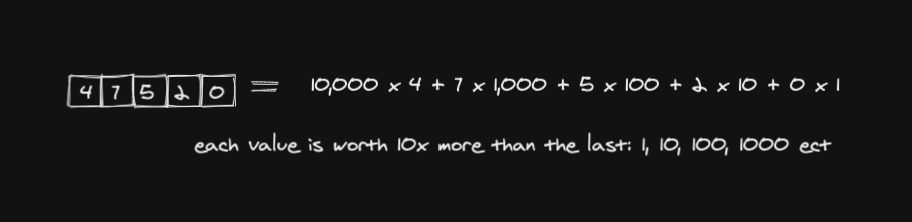If you haven't already made the correlation, the base 2 number system does the exact same thing except the place value gets doubled every time rather than being tenfold. So if I was to convert 10110 to base 10 it would look like this:

Screen Shot 2021-07-21 at 12.10.49 PM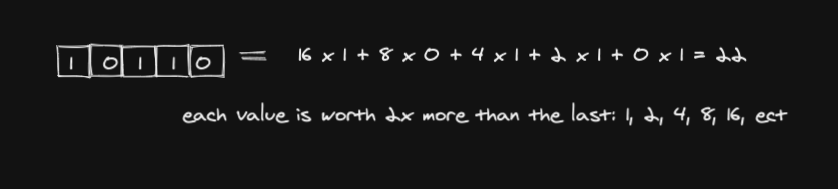So based on that we can convert any binary number using the simple pattern of multiplying the place value by 2, almost like the game 2048.

# Logic Gates

### What are logic gates?

Logic gates are little electrical components that control the logic of a computer and how it processes different electrical signals. Each and every logic gate takes a set number of binary inputs and has 1 binary output which is either powered or not. They function like building blocks for computers, just like how you would stack different-sized bricks to build a house.

### Truth Tables

Truth tables are a cool little tool that allows a person to see the output of a logic gate based on any number of combinations. Truth Tables also exist for chips which are a combination of logic gates that get packaged and can be used multiple times as a function within a program.

### AND Gate

This hopefully should be pretty self-explanatory, the output is 1 when both inputs are 1.

Truth Table:

Input 1Input 2Output
000
100
010
111

### NOT Gate

Inverts 1 input to create an output

Truth Table:

Input 1Output
01
10

### NAND Gate

Inverted version of the AND gate

Truth Table:

Input 1Input 2Output
001
101
011
110

### OR Gate

If either of the inputs or both of them are 1 the output is one

Truth Table:

Input 1Input 2Output
000
101
011
111

### NOR Gate

Inverted OR Gate

Truth Table:

Input 1Input 2Output
001
100
010
110

### XOR Gate

This one is actually somewhat complex. It takes 2 inputs and adds them while returning the sum bit as we talked about earlier in the tutorial. So when both inputs are 1 it actually returns 0 because the sum of 1 + 1 gets carried over to the next place value. Stands for EXCLUSIVE OR if anyone was wondering.

Truth Table:

Input 1Input 2Output
000
101
011
110

# Making a 4 Bit Adder

Now for the fun part, we will be using these logic gates to create a circuit that is able to add 4 bits together and represent the output. To start off, we must first build a small chip that takes in 2 bits plus a carry-in bit as an input and returns the sum bit as well as the carry-out bit. This is called a full adder and the truth table would look like this:

Input 1Input 2Carry InSum BitCarry Out
00000
00110
01010
10010
01101
1101
10101
11111

So based on what we learned from the truth table, we know that we need to add the three inputs together and output both the carry-out and the sum bit. We can start finding the sum by using 2 XOR gates which would look something like this:

Also make sure to create a subcircuit/chip by clicking the plus button next to main and to name it full adder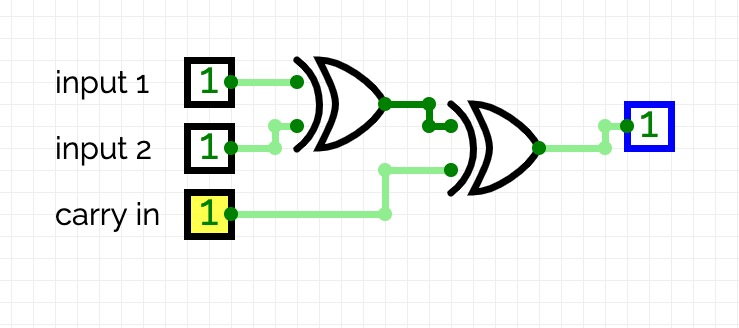In the image above, you can see that the two bits go into the XOR gate and the output of that one goes into another XOR along with the carry-in bit. This functions well, but we still haven't output the carry-out bit which is shown in the previous truth table. To do this we can add onto our current circuit like so: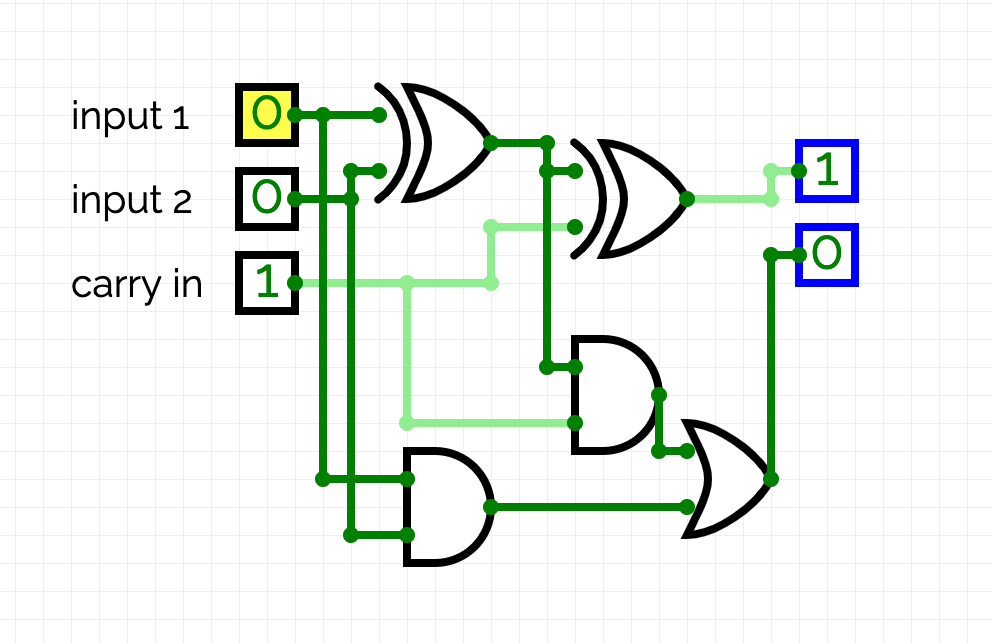This will cause the carry out to become 1 when any two or more of the inputs are one. This works because the 2 base inputs are checked as well as the sum of the 2 base inputs along with the carry-in. That is then fed into an OR gate which returns 1 if any of the AND gates returns 1.

Now we will combine multiple of these full adder chips together to add 2, 4-bit numbers together as well as accounting for a carry-in. I think it would be simpler to show the circuit and then explain it, so here it is, with the leftmost input representing the most significant place value: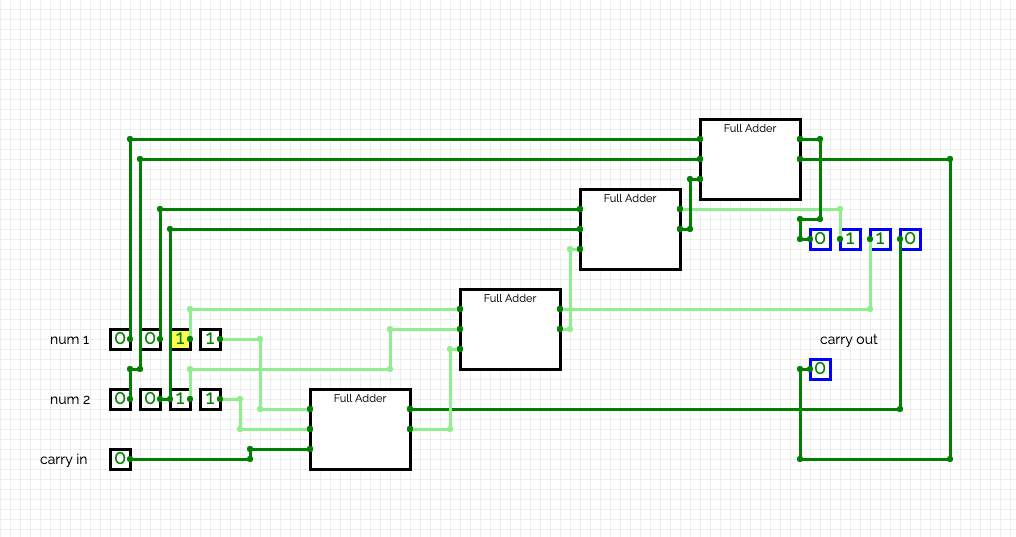Here you can see that I am adding 3 + 3 and the output is 6. This works by adding each pair of bits and linking that with the corresponding output. As for the carry-in and carry-out, they just get tacked on to the next set of full adders until it reaches the end where it is displayed.

For those of you still curious here is a link to a youtube video that explains a four-bit adder in greater detail.

# Conclusion

I would like to just take a moment and apologize if the end of this tutorial felt a bit bland as I was starting to get tired and just wanted to finish it up.

I also might continue this as it hasn't taught everything about a computer and here are a few possible future topics:

• ALU
• CPU Clock
• Memory

Anyways that is it for this tutorial and please comment with any sort of feedback.

hotnewtop
MrEconomical (2304)

this is a really awesome post, great job!

QuickV (115)

# Amazing

###### Great job :)
JWZ6 (715)

super AWESOME

I learned something

NARUTO12456789234567 (0)

When we run why does the code not display the number after "A value: "?

IMayBeMe (548)

@NARUTO12456789234567 oh that was my circuit prototyping program. It wasn’t designed to be the most user friendly, but still works. After you enter the value for input A, it should prompt you to then input a value for input B and will allow you to mess around with gates. It doesn’t output the result until you want it to